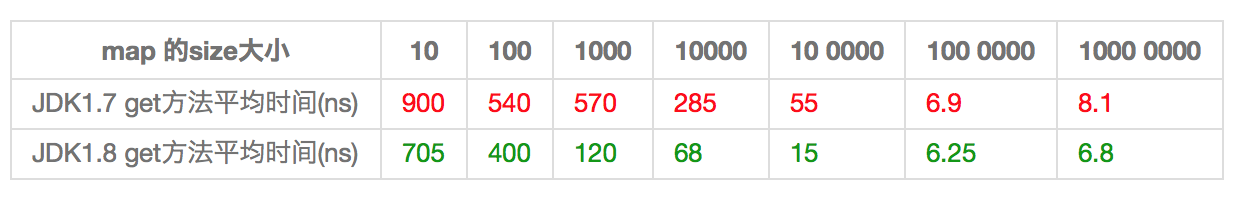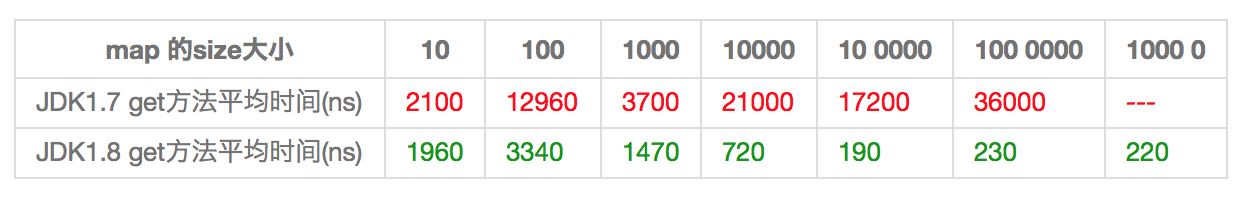# HashMap 在JDK1.8与JDK1.7的性能测试对比

JAVA 871浏览```class Key implements Comparable<Key> {
// 业余草：www.xttblog.com
private final int value;
Key(int value) {
this.value = value;
}
@Override
public int compareTo(Key o) {
return Integer.compare(this.value, o.value);
}
@Override
public boolean equals(Object o) {
if (this == o) return true;
if (o == null || getClass() != o.getClass())
return false;
Key key = (Key) o;
return value == key.value;
}
@Override
public int hashCode() {
return value;
}
}```

```public class Keys {
// 业余草：www.xttblog.com
public static final int MAX_KEY = 10_000_000;
private static final Key[] KEYS_CACHE = new Key[MAX_KEY];
static {
for (int i = 0; i < MAX_KEY; ++i) {
KEYS_CACHE[i] = new Key(i);
}
}
public static Key of(int value) {
return KEYS_CACHE[value];
}
}```

```static void test(int mapSize) {
HashMap<Key, Integer> map = new HashMap<Key,Integer>(mapSize);
for (int i = 0; i < mapSize; ++i) {
map.put(Keys.of(i), i);
}
// 业余草：www.xttblog.com
long beginTime = System.nanoTime(); //获取纳秒
for (int i = 0; i < mapSize; i++) {
map.get(Keys.of(i));
}
long endTime = System.nanoTime();
System.out.println(endTime - beginTime);
}
public static void main(String[] args) {
for(int i=10;i<= 10000000;i*= 10){
test(i);
}
}``````class Key implements Comparable<Key> {
//...
// 业余草：www.xttblog.com
@Override
public int hashCode() {
return 1;
}
}```